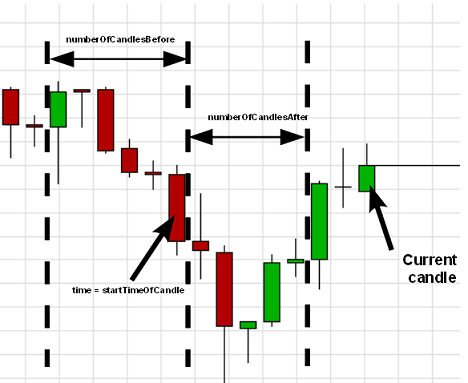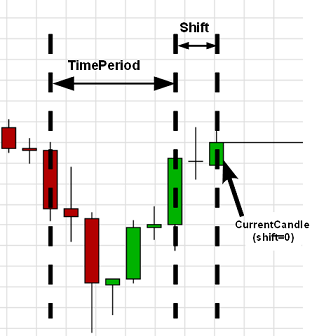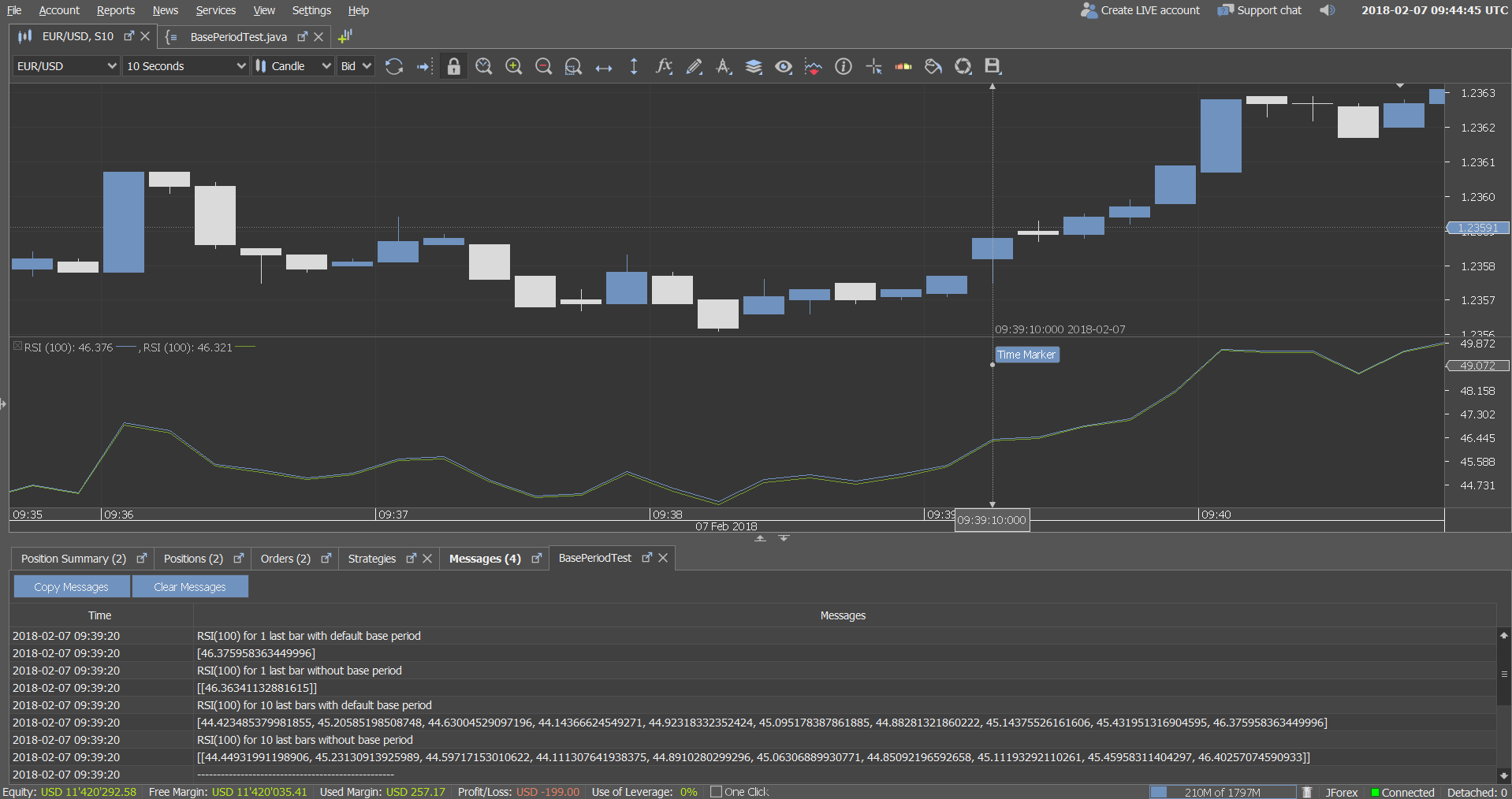# Indicator Calculation

Indicators can be calculated either by shift, time period or candle/bar period. One can divide indicator calculation approaches by source of their price inputs:

• Named indicator - base case, supported with 4 methods for each indicator (see Indicator catalog for usage examples).
• Universal methods - can be used to calculate any indicator on any type of feed
• Custom price array - indicator calculation from plain java array

There are three ways that indicator calculation interval can be specified:

• by candle interval - need to specify reference candle start time and number of candles before and after reference candle
• by time interval - need to specify start time for first and last calculated candle
• by shift - to get single indicator value

## Indicator calculation parameters

Consider IIndicators.calculateIndicator method, this method has the following parameters:

• Instrument
• Period
• OfferSide[] - Bid or Ask side of the bars
• Period - basePeriod, optional parameter
• String functionName - indicator name, this can be predefined JForex indicator or custom indicator that has been added to the JForex client or registered with it (see description here ).
• IIndicators.AppliedPrice inputTypes - type of the input data for every input that indicator requires or null if input is InputParameterInfo.Type.PRICE
• Filter filter - allows to filter candles on flats or weekends
• IIndicators.AppliedPrice
• optionalParameters - indicator optional inputs, count of which varies depending on indicator. For example, method MINMAX has 1 optional input - TimePeriod

## Calculate indicator by candle interval

• numberOfCandlesBefore - number of candles to load prior to and including the candle specified in time parameter
• time - open time of the last candle in the calculated period
• numberOfCandlesAfter - number of candles to load after and not including the candle specified in time parameterThe returned value of the method, depending on indicator output count is either:

• an array of double (if indicator has one output)
• or an array of double arrays, where first dimension stands for different output and second dimensions stands for output index

### Named method

Calculate MINMAX indicator using a named method (API reference)

``````int candlesBefore = 5;
int candlesAfter = 0;
int minMaxPeriod = 10;

long currBarTime = history.getBar(
Instrument.EURUSD, Period.ONE_MIN, OfferSide.ASK, 0)
.getTime();

Object[] minMaxResult = indicators.minMax(
Instrument.EURUSD,
Period.ONE_MIN,
IIndicators.AppliedPrice.OPEN,
minMaxPeriod,
Filter.NO_FILTER,
candlesBefore,
currBarTime,
candlesAfter);

double[][] minMax = {(double[]) minMaxResult, (double[]) minMaxResult};
int last = minMax.length - 1;

double minShift0 = minMax[last];
double maxShift0 = minMax[last];

double minShift1 = minMax[last - 1];
double maxShift1 = minMax[last - 1];

double minShift4 = minMax;
double maxShift4 = minMax;``````

NamedIndicatorMethods.java

### Universal method

To calculate MINMAX indicator using a general calculateIndicator method ( API reference ):

``````int candlesBefore = 5;
int candlesAfter = 0;
int minMaxPeriod = 10;

IFeedDescriptor feedDescriptor = new TimePeriodAggregationFeedDescriptor(
Instrument.EURUSD, Period.ONE_MIN, OfferSide.ASK, Filter.NO_FILTER);

long currBarTime = history.getFeedData(feedDescriptor, 0).getTime();

Object[] minMaxResult = indicators.calculateIndicator(
feedDescriptor,
Period.ONE_HOUR,
"MINMAX",
new AppliedPrice[] {AppliedPrice.OPEN},
new Object[] {minMaxPeriod},
candlesBefore,
currBarTime,
candlesAfter);

double[][] minMax = {(double[]) minMaxResult, (double[]) minMaxResult};
int last = minMax.length - 1;

double minShift0 = minMax[last];
double maxShift0 = minMax[last];

double minShift1 = minMax[last - 1];
double maxShift1 = minMax[last - 1];

double minShift4 = minMax;
double maxShift4 = minMax;``````

UniversalIndicatorMethods.java

## Calculate indicator by shift

• shift - number of candles back starting from the current bar. 0 - current candle(currently generated from ticks), 1 - previous candle(last formed candle), 2 - current candle minus 2 candles and so on.The returned value of the method, depending on indicator output count is either:

• a double value (if indicator has one output, like SMA)
• or an array of double, where each value stands for a different output (e.g. for MINMAX 1st value stand for min and 2nd value - for max)

### Named method

Calculate MINMAX indicator using named method (API reference)

``````int minMaxPeriod = 10;
double[] minMax = indicators.minMax(
Instrument.EURUSD,
Period.ONE_MIN,
IIndicators.AppliedPrice.OPEN,
minMaxPeriod,
3);

double min = minMax;
double max = minMax;``````

NamedIndicatorMethods.java

### Universal method

To calculate MINMAX indicator using a general calculateIndicator method ( API reference ) :

``````int minMaxPeriod = 10;

IFeedDescriptor feedDescriptor = new TimePeriodAggregationFeedDescriptor(
Instrument.EURUSD, Period.ONE_MIN, OfferSide.ASK, Filter.NO_FILTER);

Object[] minMax = indicators.calculateIndicator(
feedDescriptor,
"MINMAX",
new AppliedPrice[] {AppliedPrice.OPEN},
new Object[] {minMaxPeriod},
3);

double min = (double) minMax;
double max = (double) minMax;``````

UniversalIndicatorMethods.java

## Calculate indicator by time interval

• from - start time of the first candle in the calculated period
• to - start time of the last candle in the calculated period

The returned value of the method, depending on indicator output count is either:

• an array of double (if indicator has one output)
• or an array of double arrays, where each of the arrays (i.e. first dimension of returned two-dimensional array) stands for a different output (e.g. for MINMAX 1st array stands for array of min and 2nd value - for array of max)

### Named method

Calculate MINMAX indicator using named method (API reference)

``````int minMaxPeriod = 10;

long timeTo = history.getBar(
Instrument.EURUSD, Period.ONE_MIN, OfferSide.ASK, 1)
.getTime();

long timeFrom = history.getBar(
Instrument.EURUSD, Period.ONE_MIN, OfferSide.ASK, 5)
.getTime();

Object[] minMaxResult = indicators.minMax(
Instrument.EURUSD,
Period.ONE_MIN,
IIndicators.AppliedPrice.OPEN,
minMaxPeriod,
Filter.NO_FILTER,
timeFrom,
timeTo);

double[][] minMax = {(double[]) minMaxResult, (double[]) minMaxResult};
int last = minMax.length - 1;

double minShift0 = minMax[last];
double maxShift0 = minMax[last];

double minShift1 = minMax[last - 1];
double maxShift1 = minMax[last - 1];

double minShift4 = minMax;
double maxShift4 = minMax;``````

NamedIndicatorMethods.java

### Universal method

To calculate MINMAX indicator using a general calculateIndicator method ( API reference ) :

``````int minMaxPeriod = 10;

IFeedDescriptor feedDescriptor = new TimePeriodAggregationFeedDescriptor(
Instrument.EURUSD, Period.ONE_MIN, OfferSide.ASK, Filter.NO_FILTER);

long timeTo = history.getFeedData(feedDescriptor, 1).getTime();

long timeFrom = history.getTimeForNBarsBack(
feedDescriptor.getPeriod(),
timeTo,
5);

Object[] minMaxResult = indicators.calculateIndicator(
feedDescriptor,
Period.ONE_HOUR,
"MINMAX",
new AppliedPrice[] {AppliedPrice.OPEN},
new Object[] {minMaxPeriod},
timeFrom,
timeTo);

double[][] minMax = {(double[]) minMaxResult, (double[]) minMaxResult};
int last = minMax.length - 1;

double minShift0 = minMax[last];
double maxShift0 = minMax[last];

double minShift1 = minMax[last - 1];
double maxShift1 = minMax[last - 1];

double minShift4 = minMax;
double maxShift4 = minMax;``````

UniversalIndicatorMethods.java

## Base period

Some indicators have recurrent calculation formula - next output value depends not only on input values, but also on previously calculated output values. As a result, such indicators have unstable period - some number of additional candles must be used in calculations before we can obtain stable value of indicator. MAX(4 * Lookback, 100) additional candles are used in JForex for unstable-period indicators calculation on charts and from strategies, but calculated values still can slightly vary for the same indicator with identical parameters on the same candle, because they depend on start candle for calculations anyway. Therefore, base period was introduced in JForex to solve this problem - indicators calculation always start from first candle in corresponding base period interval (plus additional candles for unstable period before it).Attached strategy demonstrates difference in unstable-period indicators calculation with/without using of base period. On screenshot we can see that RSI(100) indicator values, calculated without using of base period for 1 candle, 10 candles (last value in array) and on chart (green line) is different for the same candle (~46.363, ~46.403 and 46.321 respectively), while with base period using we have the same value for all cases (~46.376)

BasePeriodTest.java

The information on this web site is provided only as general information, which may be incomplete or outdated. Click here for full disclaimer.